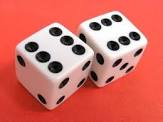# Do you play dice games?

Probability Level 4Independent trials, consisting of rolling a pair of fair dice, are performed.

What is the probability that an outcome of 5 appears before an outcome of 7?

Here, outcome of a roll is the sum of the numbers on the dices.

You can try my other Probability problems by clicking here

×